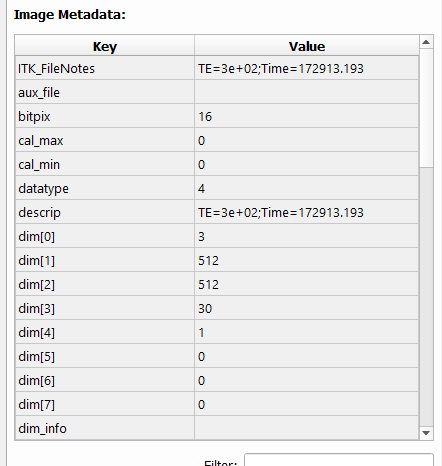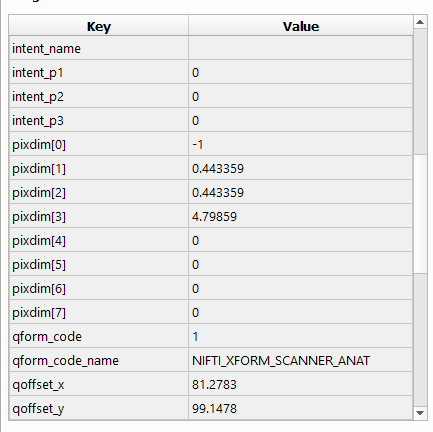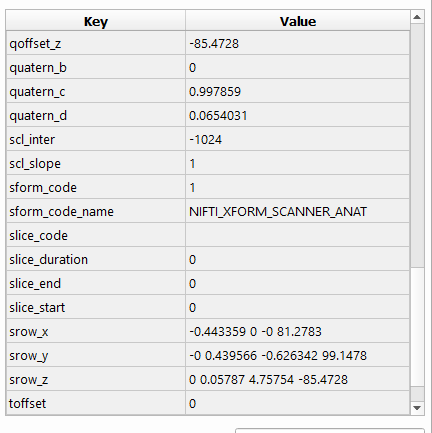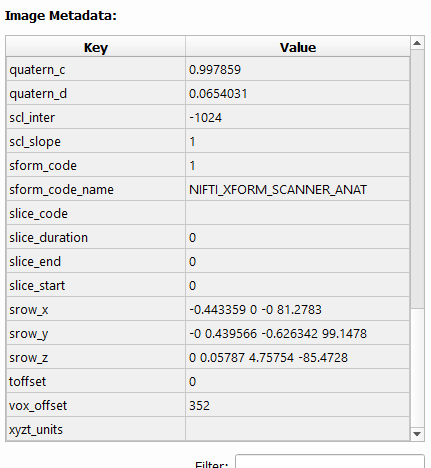Hi, I am a beginner in SimpleITK.
I used SimpleITK to load a nifti image and view its header information. The pixdim header is changed from -1 to 0. I used ITKsnap, nifti-tool and nibabel to see the header information and they all show -1. Can anyone help me with this? I don’t know why this is happening.
This is the code I used to view the header in Simpleitk

``````brain_ncct_img = sitk.ReadImage(ncct_file)
brain_ncct_data = sitk.GetArrayFromImage(brain_ncct_img)

print("({0}) = = \"{1}\"".format(k,v))
``````

This is the result I am geeting:

``````header using simpleitk
(ITK_FileNotes) = = "TE=3e+02;Time=172913.193"
(ITK_original_direction) = = "[UNKNOWN_PRINT_CHARACTERISTICS]
"
(ITK_original_spacing) = = "[UNKNOWN_PRINT_CHARACTERISTICS]
"
(aux_file) = = ""
(bitpix) = = "16"
(cal_max) = = "0"
(cal_min) = = "0"
(datatype) = = "4"
(descrip) = = "TE=3e+02;Time=172913.193"
(dim) = = "3"
(dim) = = "512"
(dim) = = "512"
(dim) = = "30"
(dim) = = "1"
(dim) = = "0"
(dim) = = "0"
(dim) = = "0"
(dim_info) = = "0"
(intent_code) = = "0"
(intent_name) = = ""
(intent_p1) = = "0"
(intent_p2) = = "0"
(intent_p3) = = "0"
(nifti_type) = = "1"
(pixdim) = = "0"
(pixdim) = = "0.443359"
(pixdim) = = "0.443359"
(pixdim) = = "4.79859"
(pixdim) = = "0"
(pixdim) = = "0"
(pixdim) = = "0"
(pixdim) = = "0"
(qform_code) = = "1"
(qform_code_name) = = "NIFTI_XFORM_SCANNER_ANAT"
(qoffset_x) = = "81.2783"
(qoffset_y) = = "99.1478"
(qoffset_z) = = "-85.4728"
(quatern_b) = = "0"
(quatern_c) = = "0.997859"
(quatern_d) = = "0.0654031"
(scl_inter) = = "-1024"
(scl_slope) = = "1"
(sform_code) = = "1"
(sform_code_name) = = "NIFTI_XFORM_SCANNER_ANAT"
(slice_code) = = "0"
(slice_duration) = = "0"
(slice_end) = = "0"
(slice_start) = = "0"
(srow_x) = = "-0.443359 0 -0 81.2783"
(srow_y) = = "-0 0.439566 -0.626342 99.1478"
(srow_z) = = "0 0.05787 4.75754 -85.4728"
(toffset) = = "0"
(vox_offset) = = "352"
(xyzt_units) = = "10"

``````

This is the header information from ITKSnap.What does SimpleITK say the voxel spacing say? Try this:

``````print(brain_ncct_img.GetSpacing())
``````

That’s what SimpleITK actually uses for spacing once the image is loaded.

It looks like this was a bug that was recently fixed in ITK

What version of SimpleITK do you have?

2 Likes

@cookpa I am using version 2.2.0

In SimpleITK 2.2.0, I see the qfac metadata that appears to be missing from your image. I’m not sure how to explain that.

``````'qfac' in brain_ncct_img.GetMetaDataKeys()
``````

returns True for me. If I write the image back out, it sets pixdim to “-1”. However, internally, the pixdim array has pixdim = 0.

The qfac is stored in pixdim, right? I don’t want to write the image. I am more concerned with the pixdim. Is there any way we can solve this?

I’m not sure I understand why `pixdim` is important outside of I/O. Once you have read the image, it has an ITK transform that can take points to and from the physical space.

Is that transform not correct for your images?

I have nifti data with header pixdim “-1” and when I read it using simpleitk and plot it using matplotlib, the image is upside down. I am guessing it has to do something with pixdim and I wanted to test theory but while reading simpleitk is making pixdim “0” and if pixdim is 0, i think we use qfac is 1.

It seems like ITK/SimpleITK might be re-doing the image orientation information. But, as Philip is asking, the orientation might work out to be the same. And if you’re using matplotlib to display an image, are you even using that orientation information?

2 Likes

I am actually using the array from this code sitk.GetArrayFromImage(brain_ncct_image) to create montage and sometimes the images in the montage is upside down but when I view it using ITK-snap it looks ok.

Accessing the image as a numpy array also ignores any orientation information. Perhaps you need to look at the orientation matrix for each image and decide if you need to flip the pixels.

1 Like

Thanks @dchen . I was able to solve the issue.

2 Likes